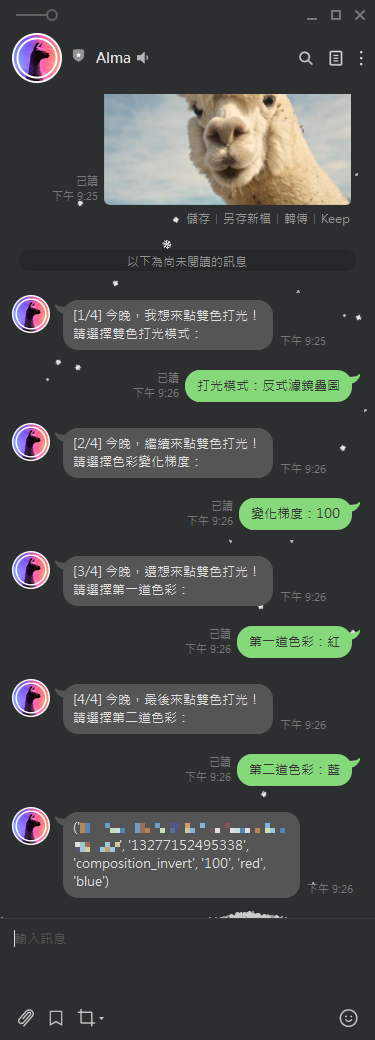0

# 第 32 天：快速回覆 QuickReply 介紹

～節錄自《聊天機器人的歷史：我媽的憂傷》

《賴田捕手：追加篇》：## 再整理資料夾

``````D:\appendix>tree /f
Folder PATH listing
Volume serial number is 9C33-6XXD
D:.
│   runtime.txt
│   requirements.txt
│   Procfile
│   Alma.py
│
└───app
__init__.py
models_for_line.py
routes.py

``````

``````D:\appendix>tree /f
Folder PATH listing
Volume serial number is 9C33-6XXD
D:.
│   runtime.txt
│   requirements.txt
│   Procfile
│   Alma.py
│
└───app
│   __init__.py
│   models_for_line.py
│   routes.py
│
└───custom_models
AlmaTalks.py
``````

• `app/custom_models/AlmaTalks.py`
``````from app import line_bot_api

from linebot.models import TextSendMessage

name = line_bot_api.get_profile(event.source.user_id).display_name
TextSendMessage(text=f"Hello {name}!")
)
``````

• `app/models_for_line.py`
``````from app import handler
from app.custom_models import AlmaTalks

from linebot.models import MessageEvent, TextMessage

def handle_message(event):
``````

## 利用 PIL 以及 numpy 完成雙色打光

1. 創造一個跟原始圖像大小一樣的雙色漸層圖像
2. 將雙色漸層圖像與原始圖像疊圖

1. 創造一個跟原始圖像大小一樣的雙色漸層圖像
``````import numpy as np

from PIL import Image
from PIL import ImageDraw

def sigmoid(x, alpha):
return 1 /(1 + np.exp(-x * alpha))

for i in range(layer_im.size):
value = sigmoid(i - layer_im.size / 2, gradient_factor / layer_im.size)
fill_color = np.array(first_tone) * value + np.array(second_tone) * (1 - value)
draw.line([(i, 0), (i, layer_im.size-1)], fill=tuple(fill_color.astype('int')))

``````

``````import matplotlib.pyplot as plt

fig, axes = plt.subplots(3, 1, figsize=(10, 10))

x = np.linspace(0, 10, 101)

for ax, g in zip(axes.flatten(), [1, 10, 100]):
ax.legend(fontsize='x-large')
``````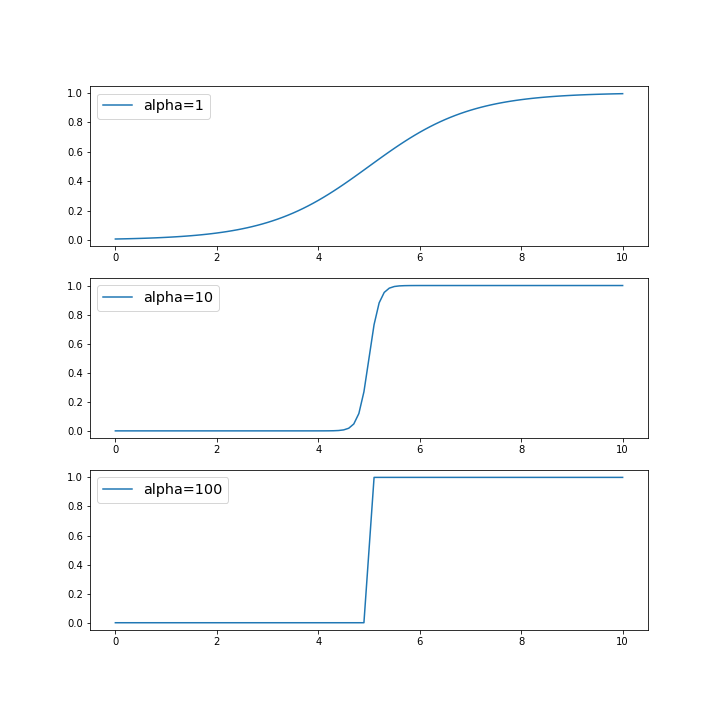`create_gradient_layer`這個函式裡，我們先用`Image.new()`來產生一張新的圖像，接著再用`ImageDraw.Draw()`為這張新的圖像上色。

• `layer_gradient = Image.new('RGB', layer_im.size)`
產生一張以`'RGB'`做為資料儲存格式的圖像`layer_gradient`，圖像大小則參考原始圖像`layer_im.size`

• `draw = ImageDraw.Draw(layer_gradient)`
準備在新的圖像`layer_gradient`上面作畫。

• `draw.line([(i, 0), (i, layer_im.size-1)], fill=tuple(fill_color.astype('int')))`
從座標`(i, 0)`開始到座標`(i, layer_im.size-1)`為止，畫一條線，該線條的顏色則由`fill=tuple(fill_color.astype('int'))`來定義。由於我們的`layer_gradient`這個新圖像是用`'RGB'`做為資料儲存的格式，因此`fill`也要用相同的形式來表示，如紅色應該表示為`(255, 0, 0)`，綠色是`(0, 255, 0)`，諸如此類。

``````create_gradient_layer(Image.open('demo_image.jpg'), (0, 255, 0), (0, 0, 255), 1)
``````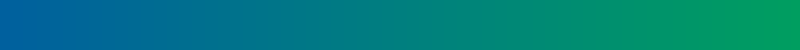``````create_gradient_layer(Image.open('demo_image.jpg'), (0, 255, 0), (0, 0, 255), 10)
``````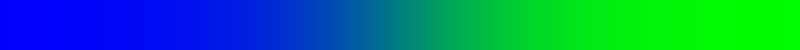``````create_gradient_layer(Image.open('demo_image.jpg'), (0, 255, 0), (0, 0, 255), 100)
``````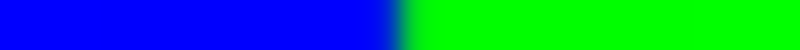1. 將雙色漸層圖像與原始圖像疊圖
這邊則可以用 PIL 提供的`blend`或是`composite`兩種方式將雙色漸層圖像與原始圖像進行疊圖。
• `Image.blend`
``````Image.blend(layer_im, layer_gradient, alpha=0.5)
``````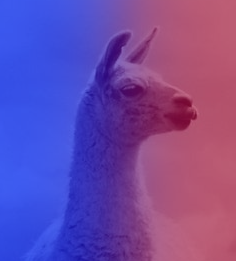• `Image.composite`
``````# 用layer_im當濾鏡，深色的地方雙色打光效果明顯

# 用ImageOps.invert(layer_im)當濾鏡，淺色的地方雙色打光效果明顯
``````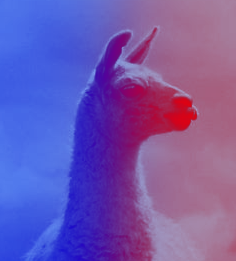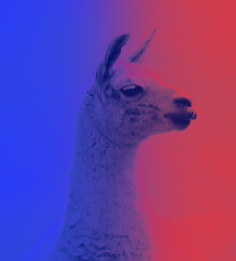``````import numpy as np
from PIL import Image
from PIL import ImageDraw
from PIL import ImageOps

def sigmoid(x, alpha):
return 1 /(1 + np.exp(-x * alpha))

for i in range(layer_im.size):
value = sigmoid(i - layer_im.size / 2, gradient_factor / layer_im.size)
fill_color = np.array(first_tone) * value + np.array(second_tone) * (1 - value)
draw.line([(i, 0), (i, layer_im.size-1)], fill=tuple(fill_color.astype('int')))

def dual_tone_run(file, mode, gradient_factor, first_tone, second_tone):
layer_im = Image.open(file).convert('RGBA')

if mode == 'blend':
elif mode == 'composite':
elif mode == 'composite_invert':
return dual_tone
``````

## 快速回覆

``````text_message = TextSendMessage(
text='Hello, world',
])
)
``````

``````quick_reply=QuickReply(
items=[
…
]
)
``````

``````action=PostbackAction(
label='postback',
display_text='postback text',
)
``````
• `label='postback'`
用來設定代表觸發該動作的按鈕的顯示文字。

• `display_text='postback text'`
觸發該動作之後，系統為使用者傳送的文字訊息。也就是說，點擊此按鈕，就好像使用者向 LINE BOT 傳送了`'postback text'`這樣的文字訊息。但跟`MessageAction`不同的是，LINE BOT 並不會因此收到`MessageEvent`。相對的，LINE BOT 會收到的是回發事件`PostbackEvent`，因為這可是`PostbackAction`啊。所以說，跟`MessageAction`不同，這邊的文字訊息只是假的，是顯示文字 (`display_text`) 而已。

• `data='action=buy&itemid=1'`
這個才是`PostbackAction`真正傳送到 LINE BOT 的資訊。LINE BOT 會收到`PostbackEvent`，而我們可以藉由`event.postback.data`來拿到這些資訊。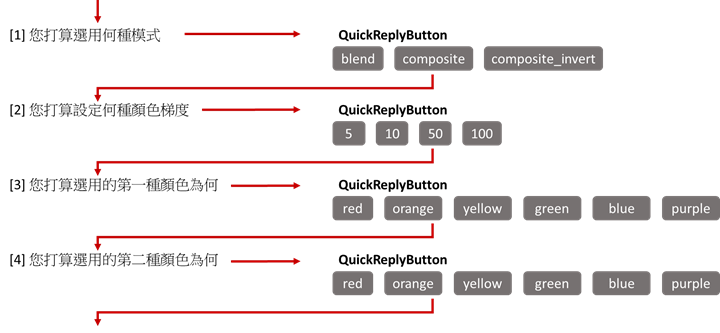• `app/models_for_line.py`
``````from app import handler
from app.custom_models import AlmaTalks

from linebot.models import ImageMessage, PostbackEvent

def handle_image(event):
AlmaTalks.phase_start(event)

def handle_postback(event):
if not event.postback.data.startswith('second_tone='):
AlmaTalks.phase_intermediate(event)
else:
AlmaTalks.phase_finish(event)
``````

• `app/custom_models/AlmaTalks.py`
``````def phase_start(event):
# 初始化表格
CallDatabase.init_table()

# 檢查使用者資料是否存在
if CallDatabase.check_record(event.source.user_id):
_ = CallDatabase.update_record(event.source.user_id, 'message_id', event.message.id)
else:
_ = CallDatabase.init_record(event.source.user_id, event.message.id)

mode_dict = {'blend': '線性疊圖', 'composite': '濾鏡疊圖', 'composite_invert': '反式濾鏡疊圖'}
TextSendMessage(
text=f"[1/4] 今晚，我想來點雙色打光！\n請選擇雙色打光模式：",
label=v,
display_text=f'打光模式：{v}',
data=f'mode={k}')) for k, v in mode_dict.items()
]
)
)
)
``````

• `app/custom_models/AlmaTalks.py`
``````def phase_intermediate(event):

color_dict = {
'red': '紅',
'orange': '橙',
'yellow': '黃',
'green': '綠',
'blue': '藍',
'purple': '紫'
}

'mode': '[2/4] 今晚，繼續來點雙色打光！\n請選擇色彩變化梯度：',
'first_tone': '[4/4] 今晚，最後來點雙色打光！\n請選擇第二道色彩：'
}

quick_button_dict = {
'mode':
action=PostbackAction(
label=i,
display_text=f'變化梯度：{i}',
data=f'gradient_factor={i}')) for i in (5, 10, 50, 100)
],
action=PostbackAction(
label=j,
display_text=f'第一道色彩：{j}',
data=f'first_tone={i}')) for i, j in color_dict.items()
],
'first_tone':
action=PostbackAction(
label=j,
display_text=f'第二道色彩：{j}',
data=f'second_tone={i}')) for i, j in color_dict.items()
]
}

user_id = event.source.user_id
postback_data = event.postback.data
current_phase = postback_data.split('=')

# 依照使用者的選擇更新資料
CallDatabase.update_record(user_id, current_phase, postback_data.split('='))
TextSendMessage(
items=quick_button_dict[current_phase]))
)
``````

• `app/custom_models/AlmaTalks.py`
``````def phase_finish(event):
user_id = event.source.user_id
postback_data = event.postback.data
current_phase = postback_data.split('=')

# 更新資料並取得最後的完整設定
record = CallDatabase.update_record(user_id, current_phase, postback_data.split('='))

TextSendMessage(text=str(record))
)
``````

## 連接 Heroku Postgres

• `app/custom_models/CallDatabase.py`
``````import os
import psycopg2

def access_database():
DATABASE_URL = os.environ['DATABASE_URL']
conn = psycopg2.connect(DATABASE_URL, sslmode='require')
cursor = conn.cursor()
return conn, cursor

def init_table():
conn, cursor = access_database()
postgres_table_query = "SELECT tablename FROM pg_catalog.pg_tables WHERE schemaname != 'pg_catalog' AND schemaname != 'information_schema'"
cursor.execute(postgres_table_query)
table_records = cursor.fetchall()
table_records = [i for i in table_records]

if 'user_dualtone_settings' not in table_records:
create_table_query = """CREATE TABLE user_dualtone_settings (
user_id VARCHAR ( 50 ) PRIMARY KEY,
message_id VARCHAR ( 50 ) NOT NULL,
mode VARCHAR ( 20 ) NOT NULL,
gradient_factor VARCHAR ( 20 ) NOT NULL,
first_tone VARCHAR ( 20 ) NOT NULL,
second_tone VARCHAR ( 20 ) NOT NULL
);"""

cursor.execute(create_table_query)
conn.commit()

return True
``````

``````CREATE TABLE user_dualtone_settings (
user_id VARCHAR ( 50 ) PRIMARY KEY,
message_id VARCHAR ( 50 ) NOT NULL,
mode VARCHAR ( 20 ) NOT NULL,
gradient_factor VARCHAR ( 20 ) NOT NULL,
first_tone VARCHAR ( 20 ) NOT NULL,
second_tone VARCHAR ( 20 ) NOT NULL
);
``````

• `app/custom_models/CallDatabase.py`
``````def check_record(user_id):
conn, cursor = access_database()

postgres_select_query = f"SELECT * FROM user_dualtone_settings WHERE user_id = '{user_id}';"

cursor.execute(postgres_select_query)
user_settings = cursor.fetchone()

return user_settings
``````

• `app/custom_models/CallDatabase.py`
``````def init_record(user_id, message_id):
conn, cursor = access_database()

table_columns = '(user_id, message_id, mode, gradient_factor, first_tone, second_tone)'
postgres_insert_query = f"INSERT INTO user_dualtone_settings {table_columns} VALUES (%s,%s,%s,%s,%s,%s)"

record = (user_id, message_id, 'blend', '50', 'red', 'blue')

cursor.execute(postgres_insert_query, record)
conn.commit()

cursor.close()
conn.close()

return record
``````

• `app/custom_models/CallDatabase.py`
``````def update_record(user_id, col, value):
conn, cursor = access_database()

postgres_update_query = f"UPDATE user_dualtone_settings SET {col} = %s WHERE user_id = %s"
cursor.execute(postgres_update_query, (value, user_id))
conn.commit()

postgres_select_query = f"SELECT * FROM user_dualtone_settings WHERE user_id = '{user_id}';"

cursor.execute(postgres_select_query)
user_settings = cursor.fetchone()

cursor.close()
conn.close()

return user_settings
``````

``````D:\appendix>tree /f
Folder PATH listing
Volume serial number is 9C33-6XXD
D:.
│   runtime.txt
│   requirements.txt
│   Procfile
│   Alma.py
│
└───app
│   __init__.py
│   models_for_line.py
│   routes.py
│
└───custom_models
AlmaTalks.py
CallDatabase.py
``````